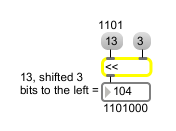# <<

Shift all bits to the left

## Description

<< has the effect of multiplying a number by a power of two, but is less expensive in computer time.

## Arguments

Name Type Opt Description
initial-value int opt Sets an initial value for the number of bits by which to shift leftward.

## Messages

 bang In left inlet: Performs the bit-shift with the numbers currently stored. If there is no argument, << initially holds 0 as the number of bits by which to shift. int input [int] In left inlet: All bits of the number, in binary form, are shifted to the left by a certain number of bits. The resulting number is sent out the outlet. (inlet1) amount-of-bitshift [int] In right inlet: The number is stored as the number of bits to left-shift the number in the left inlet. float input [float] Converted to int. set set-input [int] Sets input to the object without causing output (bang will output it). list input [list] In left inlet: The first number is bit-shifted to the left by the number of bits specified by the second number.

## Output

int: The number in the left inlet is bit-shifted to the left by a certain number of bits. The number of bits by which to shift is specified by the number in the right inlet. The output is the resulting bit-shifted number.

## ExamplesSame effect as multiplying by a power of 2